# Ricardo Borges

Personal blog## Data Structures in TypeScript - Graph

The graph is a data structure that consists of vertices (or nodes) that can be connected to other vertices by edges.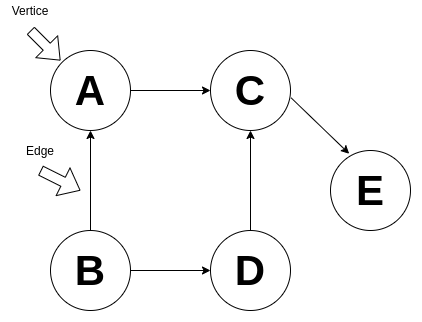The degree is the number of edges that are connected to a vertex, for example, the vertex A has a degree of 1 and the vertex C has a degree of 2.

Graphs can either directed or undirected, directed graphs are like a one-way street, undirected is like a two-way street.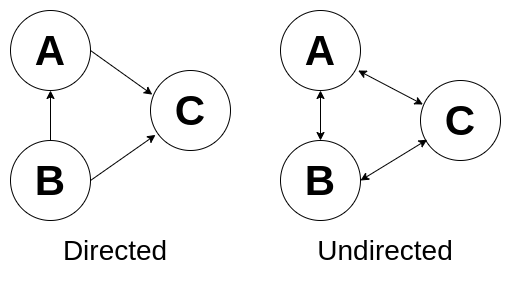Graphs can also have cycles.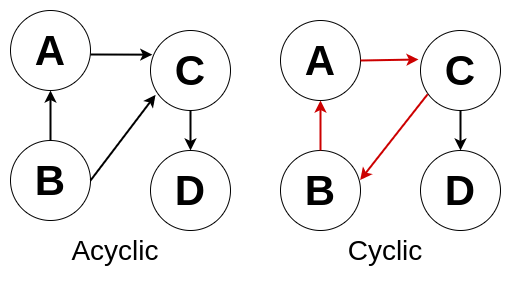Graphs might be a disconnected one, that means it consists of isolated subgraphs, or a connected one, in which all every pair of nodes are connected by an edge.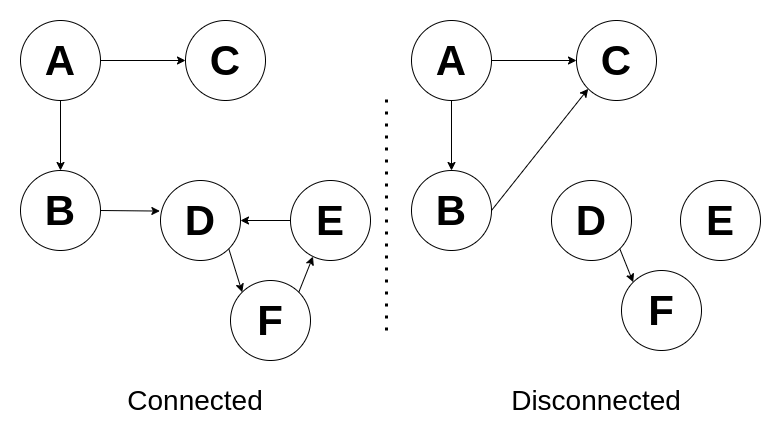Graphs can be used to represent networks, websites structure, also used in path optimization algorithms, there are applications in other fields, such as linguistics, physics, chemistry, biology, mathematics, etc.

### Representation

Graphs can be represented with

• Adjacency list - Every node stores a list of adjacent vertices, for example, an array or that contains all vertices and each vertex contains another array with adjacent vertices, other data structures can be used instead of an array, like a hash table and a linked list.

• Adjacency matrix - An NxN boolean matrix (where N is the number of vertices), if the matrixi stores the value true, there is a connection between the vertices i and j. In an undirected graph matrixj also will store the value true. You can use other types instead of boolean, for example, numbers to represent weight.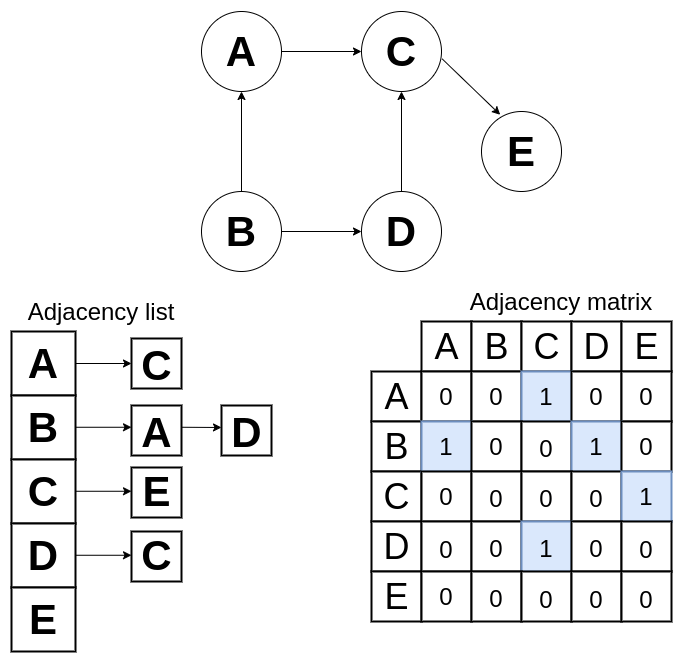### Graph Search

#### Depth-first search

Depth-first search is a way to navigate a graph, it starts from a given vertex and visits each branch completely before moving to another branch. DFS is often used when we need to visit every node in the graph.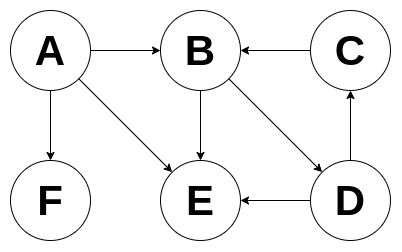Using DPS on the graph above the nodes will be visited in the following order: A, B, D, C, E, F.

This is another way to navigate a graph, it starts from a given vertex and visits all adjacent vertices before go to any of their children. BFS is useful to find a path between two nodes.Using DPS on the graph above the nodes will be visited in the following order: A, B, E, F, D, C.

#### Bidirectional search

Consists of running two breadth-first searches simultaneously, each one starts from a different vertex and runs until they collide. This is useful to find the shortest path between two vertices.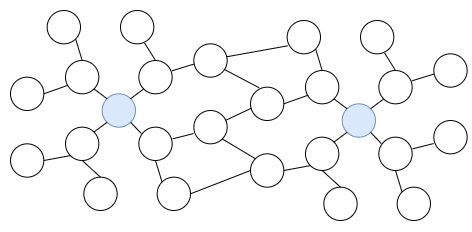Heres an implementation of a directed graph using a adjacency list, because it will perform better in almost all operations:

``````1export class Node<T> {
2  data: T;
4  comparator: (a: T, b: T) => number;
5
6  constructor(data: T, comparator: (a: T, b: T) => number) {
7    this.data = data;
8    this.adjacent = [];
9    this.comparator = comparator;
10  }
11
14  }
15
16  removeAdjacent(data: T): Node<T> | null {
17    const index = this.adjacent.findIndex(
18      (node) => this.comparator(node.data, data) === 0
19    );
20
21    if (index > -1) {
22      return this.adjacent.splice(index, 1);
23    }
24
25    return null;
26  }
27}
28
29class Graph<T> {
30  nodes: Map<T, Node<T>> = new Map();
31  comparator: (a: T, b: T) => number;
32
33  constructor(comparator: (a: T, b: T) => number) {
34    this.comparator = comparator;
35  }
36
37  /**
38   * Add a new node if it was not added before
39   *
40   * @param {T} data
41   * @returns {Node<T>}
42   */
43  addNode(data: T): Node<T> {
44    let node = this.nodes.get(data);
45
46    if (node) return node;
47
48    node = new Node(data, this.comparator);
49    this.nodes.set(data, node);
50
51    return node;
52  }
53
54  /**
55   * Remove a node, also remove it from other nodes adjacency list
56   *
57   * @param {T} data
58   * @returns {Node<T> | null}
59   */
60  removeNode(data: T): Node<T> | null {
61    const nodeToRemove = this.nodes.get(data);
62
63    if (!nodeToRemove) return null;
64
65    this.nodes.forEach((node) => {
67    });
68
69    this.nodes.delete(data);
70
71    return nodeToRemove;
72  }
73
74  /**
75   * Create an edge between two nodes
76   *
77   * @param {T} source
78   * @param {T} destination
79   */
80  addEdge(source: T, destination: T): void {
81    const sourceNode = this.addNode(source);
82    const destinationNode = this.addNode(destination);
83
85  }
86
87  /**
88   * Remove an edge between two nodes
89   *
90   * @param {T} source
91   * @param {T} destination
92   */
93  removeEdge(source: T, destination: T): void {
94    const sourceNode = this.nodes.get(source);
95    const destinationNode = this.nodes.get(destination);
96
97    if (sourceNode && destinationNode) {
99    }
100  }
101
102  /**
103   * Depth-first search
104   *
105   * @param {T} data
106   * @param {Map<T, boolean>} visited
107   * @returns
108   */
109  private depthFirstSearchAux(node: Node<T>, visited: Map<T, boolean>): void {
110    if (!node) return;
111
112    visited.set(node.data, true);
113
114    console.log(node.data);
115
116    node.adjacent.forEach((item) => {
117      if (!visited.has(item.data)) {
118        this.depthFirstSearchAux(item, visited);
119      }
120    });
121  }
122
123  depthFirstSearch() {
124    const visited: Map<T, boolean> = new Map();
125    this.nodes.forEach((node) => {
126      if (!visited.has(node.data)) {
127        this.depthFirstSearchAux(node, visited);
128      }
129    });
130  }
131
132  /**
133   * Breadth-first search
134   *
135   * @param {T} data
136   * @returns
137   */
138  private breadthFirstSearchAux(node: Node<T>, visited: Map<T, boolean>): void {
139    const queue: Queue<Node<T>> = new Queue();
140
141    if (!node) return;
142
144    visited.set(node.data, true);
145
146    while (!queue.isEmpty()) {
147      node = queue.remove();
148
149      if (!node) continue;
150
151      console.log(node.data);
152
153      node.adjacent.forEach((item) => {
154        if (!visited.has(item.data)) {
155          visited.set(item.data, true);
157        }
158      });
159    }
160  }
161
163    const visited: Map<T, boolean> = new Map();
164    this.nodes.forEach((node) => {
165      if (!visited.has(node.data)) {
167      }
168    });
169  }
170}
171
172function comparator(a: number, b: number) {
173  if (a < b) return -1;
174
175  if (a > b) return 1;
176
177  return 0;
178}
179
180const graph = new Graph(comparator);``````